# Components of a table (of a database)

## SQL Tables

A table is a collection of rows having one or more columns. A row is an instance of a row type. Every row of the same table has the same row type. The value of the n-th field of every row in a table is the value of the n-th column of that row in the table. The row is the smallest unit of data that can be inserted into a table and deleted from a table.
The number of rows in a table is its cardinality. A table whose cardinality is 0 (zero) is said to be empty.

Following image are pictorial presentation of a table and different components of it :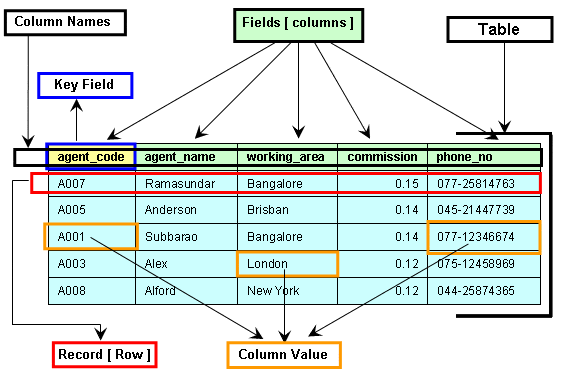A column name can be used in more than one tables and to maintain the integrity of data and reduce redundancy. This is called a relation.

## Elements of a table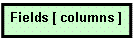The information of a table stored in some heads, those are fields or columns. Columns show vertically in a table.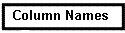Each field or column has an individual name. A table cannot contain the same name of two different columns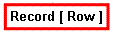All the columns in a table make a row. Each row contains all the information of individual topics.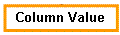The value of each field makes a row is the column value.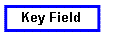Each table should contain a field which can create a link with another one or more table is the key field of a table.

Practice SQL Exercises